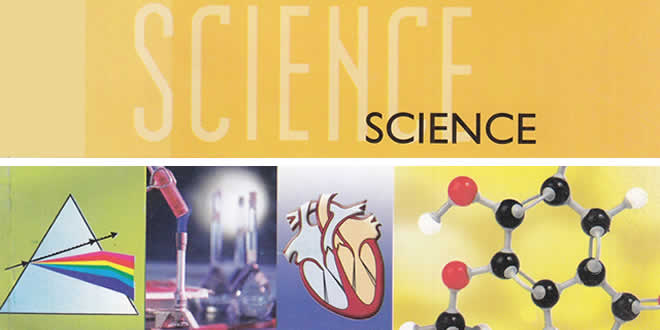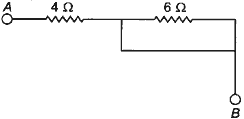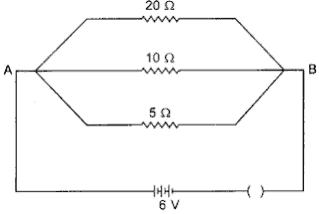Monday , August 8 2022# Electricity MCQs: 10th Class Science Chapter 12

Sources of Energy MCQs: CBSE Class 10 Science Chapter 12 Electricity Multiple Choice Questions with Answers. MCQ Questions for Class 10 Science with answers was prepared based on latest exam pattern. Students can solve NCERT Class 10 Science Electricity multiple choice questions with answers to know their preparation level.

 Class: 10th Class Subject: Science Chapter: Chapter 12: Electricity MCQs: 42 Questions Quiz: – Questions

## Electricity MCQs

### Class 10 Science Electricity MCQs with Answers & Explanation:

Free PDF Download of CBSE Class 10 Science Chapter 12 Electricity Multiple Choice Questions with Answers. MCQ Questions for Class 10 Science with Answers was Prepared Based on Latest Exam Pattern. Students can solve NCERT Class 10 Science Electricity Multiple Choice Questions with Answers to know their preparation level.

## Class 10 Science MCQs Chapter 12 Electricity

#### Question 1. Assertion: When a battery is short circuited, the terminal voltage is zero.

Reason: In short circuit, the current is zero.

(a) Both A and R are true and R is the correct explanation of A.
(b) Both A and R are true but R is not the correct explanation of A.
(c) A is true but R is false.
(d) A is false but R is true.
(e) Both A and R are false.

Answer: (c) A is true but R is false

#### Question 2. Assertion: In an open circuit, the current passes from one terminal of the electric cell to another.

Reason: Generally, the metal disc of a cell acts as a positive terminal.

(a) Both A and R are true and R is the correct explanation of A.
(b) Both A and R are true but R is not the correct explanation of A.
(c) A is true but R is false.
(d) A is false but R is true.
(e) Both A and R are false.

Answer: (d) A is false but R is true.

#### Question 3. Electrical resistivity of any given metallic wire depends upon

(a) its thickness
(b) its shape
(c) nature of the material
(d) its length

Answer: (c) nature of the material

#### Question 4. Two devices are connected between two points say A and B in parallel. The physical quantity that will remain the same between the two points is

(a) current
(b) voltage
(c) resistance
(d) None of these

(a) 30 V
(b) 10 V
(c) 20 V
(d) 25 V

(a) 10-8 Ω-m
(b) 101 Ω-m
(c) 10-6 Ω-m
(d) 106 Ω-m

(a) 440 W
(b) 110 W
(c) 55 W
(d) 0.0023 W

#### Question 8. The electrical resistance of insulators is

(a) high
(b) low
(c) zero
(d) infinitely high

#### Question 9. When electric current is passed, electrons move from:

(a) high potential to low potential.
(b) low potential to high potential.
(c) in the direction of the current.
(d) against the direction of the current.

Answer: (b) low potential to high potential

(a) copper
(b) nichrome
(c) aluminium
(d) iron

### Electricity MCQs: 10th Class Science Chapter 12

#### Question 11. Coulomb is the SI unit of:

(a) charge
(b) current
(c) potential difference
(d) resistance

(a) 1 Joule
(b) 10 Joule
(c) zero
(d) 100 Joule

#### Question 13. The effective resistance between A and B is(a) 4Ω
(b) 6Ω
(c) May be 10 Ω
(d) Must be 10 Ω

Question 14. A student says that the resistance of two wires of same length and same area of cross section is same. This statement is correct if

(a) Both wires are of different materials
(b) Both wires are made of same material and are at different temperature.
(c) Both wires are made of same material and are at same temperature.
(d) Both wires are made of different materials and are at the same temperature.

Answer: (c) Both wires are made of same material and are at same temperature.

(a) 2.5 A
(b) 5.0 A
(c) 7.5 A
(d) 10 A

(a) 100%
(b) 200%
(c) 300%
(d) 400%

(a) P
(b) P/2
(c) 2P
(d) 4P

(a) R1 < R2
(b) R2 < R1
(c) R1 = R2
(d) R1 ≥ R2

#### Question 19. A metallic conductor has loosely bound electrons called free electrons. The metallic conductor is

(a) negatively charged
(b) positively charged
(c) neutral
(d) Either positively charged or negatively charged

(a) 2
(b) 3
(c) 4
(d) 6

## Electricity MCQs: 10th Class Science Chapter 12

#### Question 21. Resistivity of a metallic wise depends on

(a) its length
(b) its shape
(c) its thickness
(d) nature of material

#### Question 22. Assertion: Conductors allow the current to flow through themselves.

Reason: They have free charge carriers.

(a) Both A and R are true and R is the correct explanation of A.
(b) Both A and R are true but R is not the correct explanation of A.
(c) A is true but R is false.
(d) A is false but R is true.
(e) Both A and R are false.

Answer: (a) Both A and R are true and R is the correct explanation of A.

#### Question 23. Assertion: Bending of wire decrease the resistance of electric wire.

Reason: The resistance of a conductor depends on length, thickness, nature of material and temperature of the conductor.

(a) Both A and R are true and R is the correct explanation of A.
(b) Both A and R are true but R is not the correct explanation of A.
(c) A is true but R is false.
(d) A is false but R is true.
(e) Both A and R are false.

Answer: (a) Both A and R are true and R is the correct explanation of A.

#### Question 24. What is the commercial unit of electrical energy?

(a) Joules
(b) Kilojoules
(c) Kilowatt-hour
(d) Watt-hour

(a) 2 A
(b) 4 A
(c) 8 A
(d) 16 A

(a) more length
(c) less length

#### Question 27. Three resistors of 1 Ω, 2 ft and 3 Ω are connected in parallel. The combined resistance of the three resistors should be

(a) greater than 3 Ω
(b) less than 1 Ω
(c) equal to 2 Ω
(d) between 1 Ω and 3 Ω

Answer: (b) less than 1 Ω

#### Question 28. Which of the following gases are filled in electric bulbs?

(a) Helium and Neon
(b) Neon and Argon
(c) Argon and Hydrogen
(d) Argon and Nitrogen

(a) resistance
(b) voltage
(c) current
(d) temperature

(a) 100 W
(b) 75 W
(c) 50 W
(d) 25 W

### Electricity MCQs: 10th Class Science Chapter 12

(a) 10 volt
(b) 1000 volt
(c) 10-3 volt
(d) 10-6 volt

(a) 1/25
(b) 1/5
(c) 5
(d) 25

#### Question 33. Electric potential is a:

(a) scalar quantity
(b) vector quantity
(c) neither scalar nor vector
(d) sometimes scalar and sometimes vector

(a) 1/5 Ω
(b) 10 Ω
(c) 5 Ω
(d) 1 Ω

(a) 1020
(b) 1016
(c) 1018
(d) 1023

(a) 48 Ω
(b) 400 Ω
(c) 484 Ω
(d) 48.4 Ω

#### Electricity MCQs – Question 37. The nature of the graph between potential difference and the electric current flowing through a conductor is

(a) parabolic
(b) circle
(c) straight line
(d) hyperbolic

#### Question 38. Two resistors are connected in series gives an equivalent resistance of 10 Ω. When connected in parallel, gives 2.4 Ω. Then the individual resistance are

(a) each of 5 Ω
(b) 6 Ω and 4 Ω
(c) 7 Ω and 4 Ω
(d) 8 Ω and 2 Ω

Answer: (b) 6 Ω and 4 Ω

#### Question 39. Resistivity of a metallic wise depends on

(a) its length
(b) its shape
(c) its thickness
(d) nature of material

#### Question 40. Calculate the current flows through the 10 Ω resistor in the following circuit.(a) 1.2 A
(b) 0.6 A
(c) 0.2 A
(d) 2.0 A

## Electricity MCQs: 10th Class Science Chapter 12

(a) < 100 Ω
(b) < 4 Ω
(c) < 1 Ω
(d) > 2 Ω

#### Electricity MCQs – Question 42. A battery of 10 volt carries 20,000 C of charge through a resistance of 20 Ω. The work done in 10 seconds is

(a) 2 × 103 joule
(b) 2 × 105 joule
(c) 2 × 104 joule
(d) 2 × 102 joule